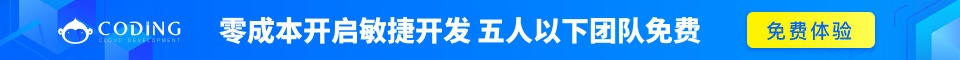# PHP array_replace() 函数完整的 PHP Array 参考手册

### 实例

<?php
\$a1=array("red","green");
\$a2=array("blue","yellow");
print_r(array_replace(\$a1,\$a2));
?>

## 定义和用法

array_replace() 函数使用后面数组的值替换第一个数组的值。

## 语法

array_replace(array1,array2,array3...)

array1必需。指定一个数组。
array2可选。指定一个要替换 array1 的值的数组。
array3,...可选。指定多个要替换 array1array2, ... 的值的数组。后面数组的值将覆盖之前数组的值。

## 技术细节

返回值： 返回被替换的数组，如果发生错误则返回 NULL。 5.3.0+

## 实例 1

<?php
\$a1=array("a"=>"red","b"=>"green");
\$a2=array("a"=>"orange","burgundy");
print_r(array_replace(\$a1,\$a2));
?>

## 实例 2

<?php
\$a1=array("a"=>"red","green");
\$a2=array("a"=>"orange","b"=>"burgundy");
print_r(array_replace(\$a1,\$a2));
?>

## 实例 3

<?php
\$a1=array("red","green");
\$a2=array("blue","yellow");
\$a3=array("orange","burgundy");
print_r(array_replace(\$a1,\$a2,\$a3));
?>

## 实例 4

<?php
\$a1=array("red","green","blue","yellow");
\$a2=array(0=>"orange",3=>"burgundy");
print_r(array_replace(\$a1,\$a2));
?>完整的 PHP Array 参考手册